## Some Remarks on Using Complex-Valued Functions

Consider functions of the form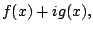(5.3)

where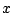is a real variable and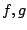are real-valued functions. For example,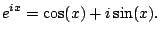We observed before that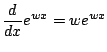hence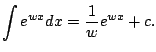For example, writing it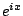as in (5.2.2), we have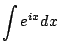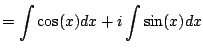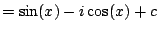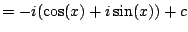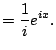Example 5.2.6   Let's compute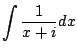. Wouldn't it be nice if we could just write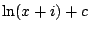? This is useless for us though, since we haven't even defined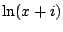! However, we can rationalize the denominator'' by writing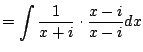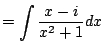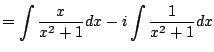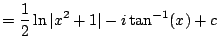This informs how we would define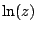for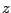complex (which you'll do if you take a course in complex analysis). Key trick: Get the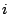in the numerator.

The next example illustrates an alternative to the method of Section 5.2.

Example 5.2.7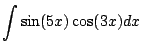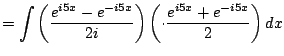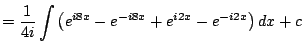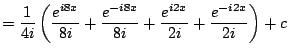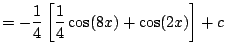This is more tedious than the method in 5.2. But it is completely straightforward. You don't need any trig formulas or anything else. You just multiply it out, integrate, etc., and remember that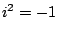.

William Stein 2006-03-15